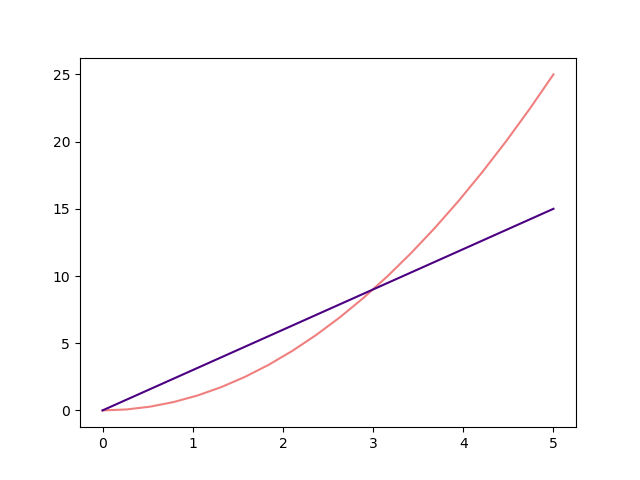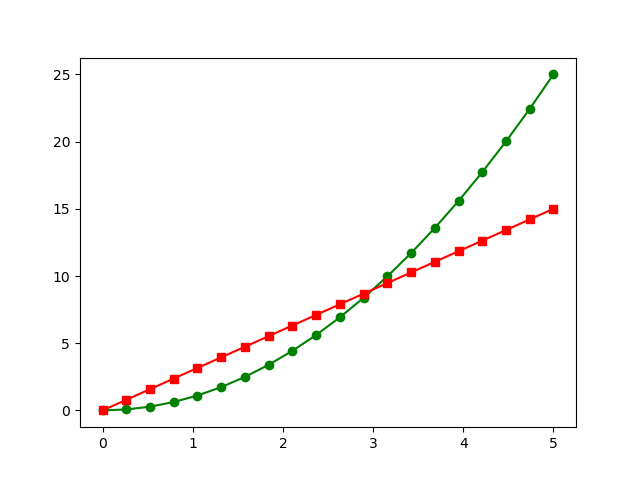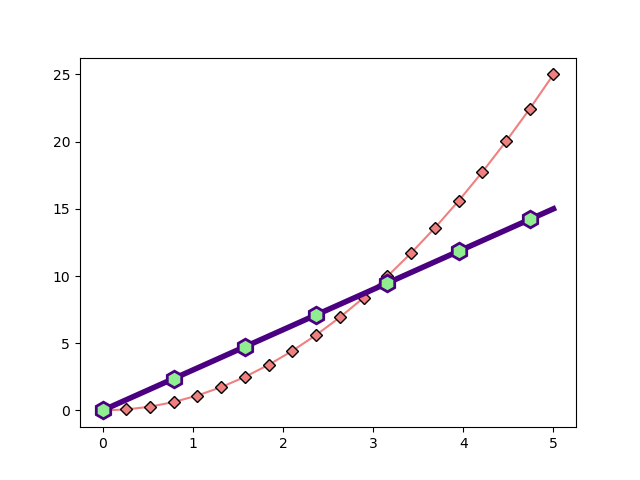# Python informer

Improve your Python coding skills

## Line plot styles in Matplotlib

Matplotlib allows you to control many aspect of your graphs. In this section we will see how to style line plots. This includes

• Controlling the colour, thickness and style (solid, dashed, dotted etc) of the lines.
• Adding markers. A marker is a small square, diamond or other shape that marks a data point.

You can choose to plot data points using lines, or markers, or both.

Matplotlib has as simple notation to set the colour, line style and marker style using a coded text string, for example “r–” creates a red, dashed line. It also supports additional parameters that give more options to control the appearance of the graph.

## Line plots

We have already seen how to create a simple line plot, using numpy to plot a function:

from matplotlib import pyplot as plt
import numpy as np

xa = np.linspace(0, 12, 100)
ya = np.sin(xa)*np.exp(-xa/4)

plt.plot(xa, ya)
plt.show()## Setting the line colour and style using a string

Matplotlib has an additional parameter to control the colour and style of the plot.

plt.plot(xa, ya 'g')


This will make the line green. You can use any colour of red, green, blue, cyan, magenta, yellow, white or black just by using the first character of the colour name in lower case (use “k” for black, as “b” means blue).

You can also alter the linestyle, for example two dashes -- makes a dashed line. This can be used added to the colour selector, like this:

plt.plot(xa, ya 'r--')


You can use “-” for a solid line (the default), “-.” for dash-dot lines, or “:” for a dotted line. Here is an example:

from matplotlib import pyplot as plt
import numpy as np

xa = np.linspace(0, 5, 20)

ya = xa**2
plt.plot(xa, ya, 'g')

ya = 3*xa
plt.plot(xa, ya, 'r--')

plt.show()As you can see, we have added two plots simply by calling plt.plot twice. You can also add multiple plots by adding them all to the same call, like this:

plt.plot(xa, xa**2, 'g', xb, 3*xa, 'r--')


## Setting the line colour and style with parameters

You might find the options above slightly limited. For example, what if you wanted different shades of green, or thicker lines?

The plt.plot function has a collection of optional parameters. Here are some of the main ones.

### Color

color takes a string. The options are:

• Single character colors, as above, eg “r”, “g” etc
• CSS colour names. There are 140 shades, with names such as seagreen, steelblue, tomato, and so on. They are listed here, but for Matplotlib the names should be written in lower case.
• RGB colours. These take the form “#rrggbb” which indicates the colour as a six digit hex value indicating the amount of red, green and blue each as a 2 digit hex value (00 to FF), again in the same way that HTML colours are specified in hex.

Here is an example:

from matplotlib import pyplot as plt
import numpy as np

xa = np.linspace(0, 5, 20)

ya = xa**2
plt.plot(xa, ya, color='lightcoral')

ya = 3*xa
plt.plot(xa, ya, color='#4b0082')  #A shade of purple

plt.show()### Line styles

You can set the width of the plot line using the linewidth parameter. For the default plot the line width is in pixels, so you will typically use 1 for a thin line, 2 for a medium line, 4 for a thick line, or more if you want a really thick line.

You can set the line style using the linestyle parameter. This can take a string such as “–”, “-.” etc, the same as the style string above.

Alternatively it can take a structure like this:

(offset, (on, off, on, off...))


The first value, offset, is the length before the pattern starts. This will usually be zero. The second parameter is a tuple of on/off values. So for example, if the value is (5, 2, 1, 2) the line will consist of a dash of 5 units, a gap of 2 units, a dash of 1 unit, a gap of 2 units. This pattern repeats for the length of the line. A “unit” in this case is equal to the width of the line.

Here is the same plot but with thicker, dashed lines, using the two different ways of defining dashes:

from matplotlib import pyplot as plt
import numpy as np

xa = np.linspace(0, 5, 20)

ya = xa**2
plt.plot(xa, ya, color='lightcoral', linewidth=4, linestyle=':')

ya = 3*xa
plt.plot(xa, ya, color='#4b0082', linewidth=6,
linestyle=(0, (5, 2, 1, 2)), dash_capstyle='round')

plt.show()


Notice that the second plot also has dash_capstyle set to “round”. This means that instead of being rectangular, each dash has rounded ends. You can see this on the purple plot.## Markers

A marker is a symbol such as a symbol such as a small dot, square, diamond etc that indicates a data point on the graph. As with lines, markers can be controlled by a simple text string, or by a set of parameters that give more options.

### Controlling markers with a string

In this example, we have added markers to our two curves:The green curve has circle markers, the red curve has square markers.

The markers appear at the data points we have defined for the plot. Since we have used np.linspace to define 20 equally spaced points between x=0 and x=5, we see 20 markers at these positions.

Here is the code:

from matplotlib import pyplot as plt
import numpy as np

xa = np.linspace(0, 5, 20)

ya = xa**2
plt.plot(xa, ya, 'g-o')

ya = 3*xa
plt.plot(xa, ya, 'r-s')

plt.show()


All we have changed from the original red and green curve at the start of the article are the style strings. “g-o” specifies a green curve, a solid line and a circle marker (the letter o). “r-s” specifies a red curve, a solid line, and a square marker (the letter s).

Notice that you must specify a line style, otherwise no line will be drawn (just the markers). You can used other line styles, for example “g-.o” for dash-dot lines.

Matplotlib supports quite a few marker shapes, here are some of the common ones:

• o - circle
• s - square
• v, <, >, ^ - triangles pointing down, left, right, up
• d, D - thin or thick diamond
• x, X - cross (x is line only, X is filled shape)
• h - hexagon

There are others and ways to defining your own shapes, refer to the Matplotlib documentation for details.

### Controlling markers with parameters

Here is a plot that uses some more advanced formatting:Here is the code:

from matplotlib import pyplot as plt
import numpy as np

xa = np.linspace(0, 5, 20)

ya = xa**2
plt.plot(xa, ya, color='lightcoral', marker='D', markeredgecolor='black')

ya = 3*xa
plt.plot(xa, ya, color='#4b0082', linewidth=4,
marker='h', markerfacecolor='lightgreen', markeredgewidth=2,
markersize=12, markevery=3)

plt.show()


For the coral coloured plot, we use the following parameters:

• marker='D' - sets the shape to a diamond. Even when using parameters, Matplotlib still uses letter codes for marker shapes.
• markeredgecolor='black' - this sets the outline of the marker to black. The marker is still filled with the default colour (ie the line colour).

For the purple plot we use the following parameters:

• marker='h' - sets the shape to a hexagon.
• markerfacecolor='lightgreen' - sets the fill colour if the marker to light green. The outline is still purple the line colour) because we aren’t using markeredgecolor to set it. If you wish, you can set the fill and outline colours.
• markeredgewidth=2 - this sets the thickness of the outline of the marker.
• markersize=12 - this sets the size of the marker.
• markevery=3 - rather than drawing a marker for every single data point, this parameter means that a marker will only be drawn every nth data point (in this case, every third point).